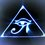# Exponent OperationsMultiplying Exponents With the Same Bases

When multiplying exponents with like bases you simply add the exponents. The base does not change.

$a^{b} \times a^{c} = a^{b + c}$

Here's an example to show why this works.

$3^{3} \times 3^{2}$

$=3 \times 3 \times\ 3 \times 3 \times 3$

$=3^5$

This is categorized under the product rules for exponents.

Dividing Exponents With the Same Bases

When dividing exponents with the same base you subtract the exponents. The base does not change.

$a^{b} \div a^{c} = a^{b - c}$

Here's an example to show why this works.

$3^{4} \div 3^{2}$

$=3 \times 3 \times\ 3 \times 3 \div 3 \div 3$

$=3 \times 3$

$=3^2$

This is categorized under the quotient rules for exponents

Exponents to Exponents

When you have a given base $a$ raised to an exponent $b$ in parentheses which is in turn raised to another exponent $c$, or $(a^{b})^{c}$ you can multiply the two exponents $b$ and $c$ and the base stays the same. In other words $(a^{b})^{c}$= $a^{b \times c}$

Although you can use this to simplify an exponent to an exponent such as

$(9^{2})^{3}$= $9^{2 \times 3}$ = $9^{6}$

Keep in mind that this works in reverse as well allowing you to factor out numbers from the exponents if necessary.

Be careful though because if there are no parentheses you must use a different rule.

When you have a given base $a$ raised to an exponent $b$ which is in turn raised to another exponent $c$, or $a^{b^{c}}$ you solve $b$ to the exponent $c$ first and the base stays the same. In other words $a^{b^{c}}$= $a^ {(b^{ c})}$

for example $9^{2^{3}}$= $9^ {(2^ {3})}$ = $9^{8}$

These are called the power rules of exponents.

Different Bases Same Exponents

The Product Rule

$a^{n} \times b^{n} = (a \times b)^{n}$

The Quotient Rule

$a^{n} \div b^{n} = (a \div b)^{n}$

Factoring out bases

This is basically just the previous section reworded because this is a very important concept. If you have a base that is not a prime number you can divide factors out of it. Lets say we have a base $d$ such that $d=a \times b$ , then it is also true that $d^{c} = a^{c} \times b^{c}$

example 1

$27^{3}$

$= (9 \times 3)^{3}$

$=9^{3} \times 3^{3}$

example 2

$=\sqrt{45}$

$=\sqrt{9 \times 5}$

$=\sqrt{9} \times \sqrt{5}$

$=3 \times \sqrt{5}$

I do not know if there is a general rule for adding exponents I find the best way is to treat each problem uniquely. That being said using the exponent rules up above you can start to see different methods which allow you to simplify these types of expressions.

example 1

$27^{2} + 9^{2}$

$= (9 \times 3)^{2} + 9^{2}$

$= 9^{2} \times 3^{2} + 9^{2}$

$= 9^{2} \times 9 + 9^{2}$

$= 9(9^{2})+ 1(9^{2})$

$= 10 (9^{2})$

This can be further simplifed.

$= 10 ((3^{2})^{2} )$

$= 10 (3^{2 +2 })$

$= 10(3^{4})$

example 2

$5 \sqrt{44} + 2 \sqrt{11}$

$= 5 \sqrt{4 \times 11} + 2 \sqrt{11}$

$= 5\times \sqrt{4} \times \sqrt{11} + 2 \sqrt{11}$

$=(5 \times 2) \sqrt{11} + 2 \sqrt{11}$

$= 10 \sqrt{11} + 2 \sqrt {11}$

$= 12 \sqrt{11}$Note by Brody Acquilano
6 years, 3 months ago

This discussion board is a place to discuss our Daily Challenges and the math and science related to those challenges. Explanations are more than just a solution — they should explain the steps and thinking strategies that you used to obtain the solution. Comments should further the discussion of math and science.

When posting on Brilliant:

• Use the emojis to react to an explanation, whether you're congratulating a job well done , or just really confused .
• Ask specific questions about the challenge or the steps in somebody's explanation. Well-posed questions can add a lot to the discussion, but posting "I don't understand!" doesn't help anyone.
• Try to contribute something new to the discussion, whether it is an extension, generalization or other idea related to the challenge.

MarkdownAppears as
*italics* or _italics_ italics
**bold** or __bold__ bold
- bulleted- list
• bulleted
• list
1. numbered2. list
1. numbered
2. list
Note: you must add a full line of space before and after lists for them to show up correctly
paragraph 1paragraph 2

paragraph 1

paragraph 2

[example link](https://brilliant.org)example link
> This is a quote
This is a quote
    # I indented these lines
# 4 spaces, and now they show
# up as a code block.

print "hello world"
# I indented these lines
# 4 spaces, and now they show
# up as a code block.

print "hello world"
MathAppears as
Remember to wrap math in $$ ... $$ or $ ... $ to ensure proper formatting.
2 \times 3 $2 \times 3$
2^{34} $2^{34}$
a_{i-1} $a_{i-1}$
\frac{2}{3} $\frac{2}{3}$
\sqrt{2} $\sqrt{2}$
\sum_{i=1}^3 $\sum_{i=1}^3$
\sin \theta $\sin \theta$
\boxed{123} $\boxed{123}$